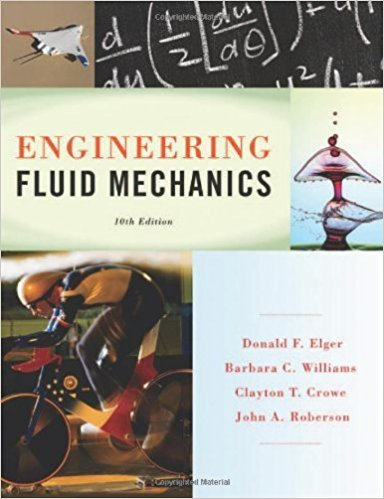×
×

# Suppose that glycerin is flowing (T = 20C) and that the pressure gradient dpldx is - 1.6ISBN: 9781118164297 288

## Solution for problem 2.33 Chapter 2

Engineering Fluid Mechanics | 10th Edition

• Textbook Solutions
• 2901 Step-by-step solutions solved by professors and subject experts
• Get 24/7 help from StudySoup virtual teaching assistantsEngineering Fluid Mechanics | 10th Edition

4 5 1 390 Reviews
30
3
Problem 2.33

Suppose that glycerin is flowing (T = 20C) and that the pressure gradient dpldx is - 1.6 kN/m3 What are the velocity and shear stress at a distance of 12 mm from the wall if the space B between the walls is 5.0 em? What are the shear stress and velocity at the wall? The velocity distribution for viscous flow between stationary plates is I dp u =---(By- y 2 ) 2f.L dx

Step-by-Step Solution:
Step 1 of 3
Step 2 of 3

Step 3 of 3

##### ISBN: 9781118164297

Unlock Textbook Solution